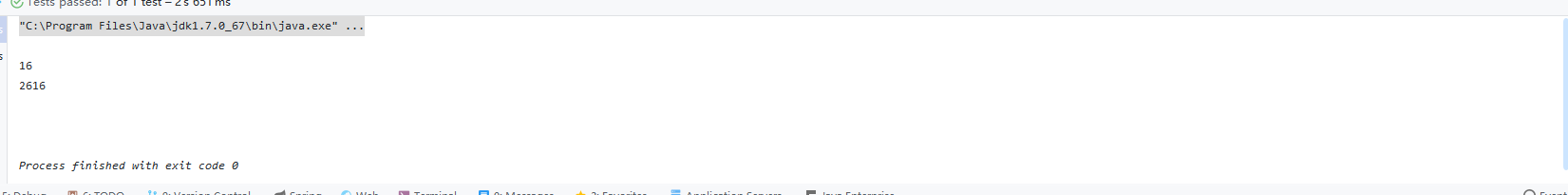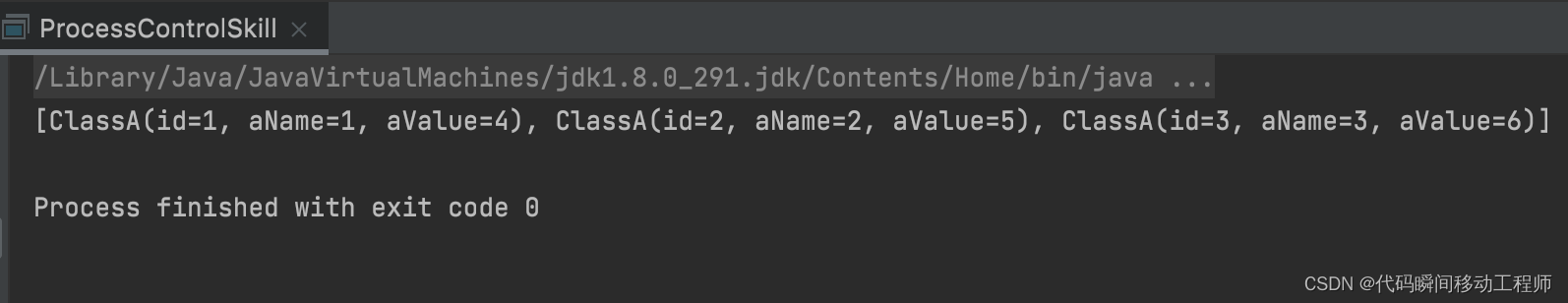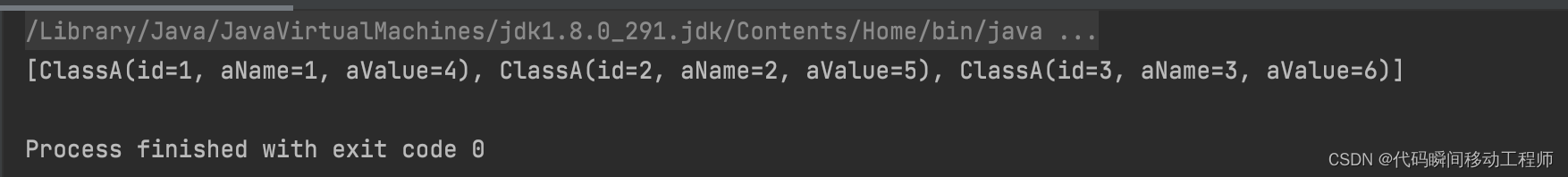• 2018-12-19 09:16:41

# 简述

循环次数较多，循环层数较多时，程序效率问题非常明显。优化后的多重for循环可以提升大半的效率。

# 方法

一、实例化变量尽量放在for循环体外，只实例化一次。
二、普通变量改为寄存器变量，如i++改为++i。前置递增运算避免了不必要的工作，它把值加1后直接返回改变了运算对象本身。
三、条件比较使用<要快于<=,同理>要快于<=。
四、把外层可以计算的尽可能放到外层；有判断条件的语句与循环不相关的操作语句尽量放在for外面。
五、应将最长的循环放在最内层，循环次数最少的放在最外层，以减少CPU跨切循环层的次数。

for (row = 0; row < 100; ++row)
{
for (col =0; col < 5; ++col）
{
sum = sum + a[row][col];
}
}


如上述代码，效率低。把row放在内层，可以提高效率。
六、对于一个可结合和可交换的合并操作来说，可以通过将一组合并操作分割成2个或更多的部分，并在最后合并结果来提高性能。
原理是–普通代码只能利用CPU的一个寄存器，分割后可以利用多个寄存器。当分割到达一个数量时，寄存器用完，性能不再提升，甚至会开始下降。代码示例如下：

//一般情况代码
for (int i = 1; i < n+1; ++i)
{
res = res OPER i;
}
//循环分割后代码
for (int i = 1; i < n; i+=2)
{
res1 = res1 OPER i;
res2 = res2 OPER (i+1);
}


测试发现，采用这个方法，浮点数计算性能的提升，明显大于整数；乘法计算性能提升，略大于加法。

# 写在最后

更多相关内容
• ## 多重循环优化

千次阅读 2016-03-30 11:41:08
上面这个代码，虽然我试了几次都没有超时，最大的耗时是2800ms，可是毕竟是4重循环，虽然实际为O(n^2)，但是参考了书上的代码进行如下优化： //枚举+二分搜索优化 import java.util.Arrays; import java.util....

四平方和 (程序设计)
四平方和定理，又称为拉格朗日定理：
每个正整数都可以表示为至多4个正整数的平方和。
如果把0包括进去，就正好可以表示为4个数的平方和。
比如：
5 = 0^2 + 0^2 + 1^2 + 2^2
7 = 1^2 + 1^2 + 1^2 + 2^2
（^符号表示乘方的意思）
对于一个给定的正整数，可能存在多种平方和的表示法。
要求你对4个数排序：
0 <= a <= b <= c <= d
并对所有的可能表示法按 a,b,c,d 为联合主键升序排列，最后输出第一个表示法
程序输入为一个正整数N (N<5000000)
要求输出4个非负整数，按从小到大排序，中间用空格分开
例如，输入：
5
则程序应该输出：
0 0 1 2
再例如，输入：
12
则程序应该输出：
0 2 2 2
再例如，输入：
773535
则程序应该输出：
1 1 267 838
资源约定：
峰值内存消耗（含虚拟机） < 256M
CPU消耗 < 3000ms
请严格按要求输出，不要画蛇添足地打印类似：“请您输入…” 的多余内容。

这道题，我当时就是写了一个四重循环遍历搜索，也跑了最大的一组样例，没有超时，就直接交了！后来，在网上发现，这样做好像是超时的。唉，还是自己做题做的少，算法研究的少啊！要是想在任何一门比赛中得到好成绩，还是需要算法过硬才行啊！

//1.四重循环直撸
import java.util.Scanner;

public class Main {

public static int N = 0;

public static void Op(){
for(int a=0;a*a<N;a++){
for(int b=0;b*b<N;b++){
for(int c=0;c*c<N;c++){
for(int d=0;d*d<N;d++){
if(a*a+b*b+c*c+d*d==N){
System.out.println(a+" "+b+" "+c+" "+d);
return ;
}
}
}
}
}
}

public static void main(String[] args) {
// TODO Auto-generated method stub
Scanner in = new Scanner(System.in);
while(in.hasNext()){
N = in.nextInt();
//long t1 = System.currentTimeMillis();
Op();
//long t2 = System.currentTimeMillis();
//System.out.println("Time cost:"+(t2-t1));
}
}

}


上面这个代码，虽然我试了几次都没有超时，最大的耗时是2800ms，可是毕竟是4重循环，虽然实际为O(n^2)，但是参考了书上的代码进行如下优化：

//枚举+二分搜索优化
import java.util.Arrays;
import java.util.Random;
import java.util.Scanner;

public class Main {

public static int n, line;
public static int[] arr;

public static void find(){
arr = new int[line];
for(int i=0;i<line;i++) arr[i] = i * i;
for(int a=0;a<line;a++){
for(int b=0;b<line;b++){
for(int c=0;c<line;c++){
int t = n - arr[a] - arr[b] - arr[c];
int index = -1;
index = Arrays.binarySearch(arr, t);
if(index>=0){
//  System.out.println("n:"+n);
System.out.println(a+" "+b+" "+c+" "+(int)Math.sqrt(t));
return ;
}
}

}
}
}

public static void main(String[] args) {
// TODO Auto-generated method stub
Scanner in = new Scanner(System.in);
//  Random rand = new Random();
for(int i=0;i<10000;i++){
//n = rand.nextInt(5000000);
n = in.nextInt();
long t1 = System.currentTimeMillis();
line = (int)Math.sqrt(n)+10;
find();
long t2 = System.currentTimeMillis();
//          System.out.println("Time cost:"+(t2-t1));
//          if(t2-t1>3000){
//              System.out.println("breakpoint!");
//              break;
//          }
}
}

}


优化后的算法复杂度从O（n^4)变为O(n^3*log n)，原理是这样的，既然是求 a^2 + b^2 + c^2 + d^2 = N，先枚举出[0，sqrt(N)+10]（加上10是为了保险）所有值的平方，并将其存在arr数组中(arr[i] = i * i)，然后问题就变成了“在数组arr中是否存有四个数A,B,C,D使得A+B+C+D=N”，将等式变形D=N-A-B-C，就是等价于在数组arr中寻找有没有t=N-A-B-C这样一个值，由于数组arr是升序的，可以直接使用二分查找，其复杂度为log n，再加上外围的三层循环，总复杂度为O(n^3*log n)。

展开全文算法 c语言
• List,String>> list = new ArrayList(); //造数据 for(int i = 0;i;i++){ Map,String> m = new HashMap(); list.add(m);...利用map来进行获取 获取数据 效率要高于使用循环处理 尽量避免在循环中运算
  List<Map<String,String>> list = new ArrayList<>();
//造数据
for(int i = 0;i<20000;i++){
Map<String,String> m = new HashMap<>();
m.put("count","test"+i);
}
//map 方式
long start = System.currentTimeMillis();
Map<String,String> map = new HashMap<>();
for(Map<String,String> m:list){
map.put(m.get("count"),m.get("count"));
}
for(Map<String,String> m:list){
map.get(m.get("count"));
}
long end = System.currentTimeMillis();
System.out.println(end-start);
// list 方式取值
start = System.currentTimeMillis();
for(Map<String,String> m:list){
for(Map<String,String> m1:list){
if(m1.get("count").equals(m.get("count"))){
m1.get("count");
break;
}
}
}
end = System.currentTimeMillis();
System.out.println(end-start);List<Map<String,String>> list = new ArrayList<>();
for(int i = 0;i<2000000;i++){
Map<String,String> m = new HashMap<>();
m.put("count","test"+i);
}
long start = System.currentTimeMillis();
int len =  list.size();
List<String> str = new ArrayList<>();
final  boolean b =  str.contains("1");
for (int i = 0; i < len; i++) {
if(b){
list.get(i).get("count");
}
}
long end = System.currentTimeMillis();
System.out.println(end-start);
start = System.currentTimeMillis();

for (int i = 0; i < len; i++) {
if(str.contains("1")){
list.get(i).get("count");
}
}
end = System.currentTimeMillis();
System.out.println(end-start);

Connected to the target VM, address: '127.0.0.1:60132', transport: 'socket'

47
39

Disconnected from the target VM, address: '127.0.0.1:60132', transport: 'socket'

Process finished with exit code 0



总结 利用map来进行获取 获取数据 效率要高于使用循环处理
尽量避免在循环中运算

展开全文• 编程技巧——多重for循环优化 目录编程技巧——多重for循环优化前言一、优化方案二、示例1.初始化数据2.双重for循环3.list结合map总结 前言 今天群友问了一个问题，多重for循环怎么优化？ 然后有感而发，将平时...

# 前言

今天群友问了一个问题，多重for循环怎么优化？
然后有感而发，将平时编码中对于该种问题的解决方法分享出来，欢迎大家一起探讨学习！

# 一、优化方案

通过list结合map的方式降低时间复杂度，达成多层for循环的优化，文中示例为即兴编写，实际编码过程中还需要根据实际业务进行参考优化。

# 二、示例

## 1.初始化数据

业务场景初步定为根据对象A和对象B的某个字段进行匹配然后更新A对象的数据。

代码如下（示例）：

public class ProcessControlSkill {

public static void main(String[] args) {
List<ClassA> aList = new ArrayList<>();
ClassA.ClassABuilder aBuilder = ClassA.builder();
aBuilder.id(1).aName("1").aValue("1");
aBuilder.id(2).aName("2").aValue("2");
aBuilder.id(3).aName("3").aValue("3");

List<ClassB> bList = new ArrayList<>();
ClassB.ClassBBuilder bBuilder = ClassB.builder();
bBuilder.id(4).bName("1").bValue("4");
bBuilder.id(5).bName("2").bValue("5");
bBuilder.id(6).bName("3").bValue("6");
}
}

@Data
@Builder
class ClassA{
private Integer id;
private String aName;
private String aValue;
}

@Data
@Builder
class ClassB{
private Integer id;
private String bName;
private String bValue;
}


## 2.双重for循环

代码如下（示例）：

        for (ClassA a : aList) {
for (ClassB b : bList) {
if (a.getAName().equals(b.getBName())){
a.setAValue(b.getBValue());
}
}
}
System.out.println(aList);


运行结果（示例）：## 3.list结合map

代码如下（示例）：

        Map<String, ClassB> bMap = bList.stream().collect(Collectors.toMap(ClassB::getBName, Function.identity()));
for (ClassA a : aList) {
ClassB b = bMap.get(a.getAName());
if (Objects.nonNull(b)){
a.setAValue(b.getBValue());
}
}
System.out.println(aList);


运行结果（示例）：## 总结

由示例可以看出，两种写法运行结果是一样的，但是从时间复杂度的角度来看，双重for循环的时间复杂度为n^2，list结合map的方式时间复杂度为2n=n。如果是3层，多层呢，时间复杂度又会再上一个层级，所以合理的利用map去优化多层for循环可以有效的降低时间复杂度。

展开全文java 开发语言
• ## 多重for循环优化，提升运行效率

万次阅读 多人点赞 2018-07-18 09:37:08
循环次数较少的时候一般...客户体验会非常不好，才研究这个情况的，事实证明，优化后的多重for循环提升了一大半的效率，是不是很神奇。 当然，本文也有借鉴其他同胞的方法。 实例化变量放在for循环外，减少实...提升效率
•   最近工作中遇到一个难题，优化一个项目的计算时间。最初，建立项目时用户少，中间使用了for循环，还是嵌套的，共两层，项目整体运行一次耗时1-2个小时。最近，随着用户量增长，项目耗时达到6-7个小时。显然是不...
• /*** @program: cwl-performance-optimization* @description: 测试for循环-嵌套循环* @author: ChenWenLong* @create: 2019-11-22 11:27**/public class TestNestedLoop {// 当需要嵌套循环时 外层循环越小 性能越...
• 主要介绍了Java for循环性能优化实现解析,文中通过示例代码介绍的非常详细，对大家的学习或者工作具有一定的参考学习价值,需要的朋友可以参考下
• merra_data是dataframe格式，按月份、小时、列进行循环，但是速度较慢，不知道应该如何将for循环代码改进，x'x ` for i in range(1,13): temp1 = merra_data_val[merra_data_val['Month']==i] temp2 = ...python
• 对于这样的多重循环，如果里面包含语句，我们都可以从循环次数判断出代码2比代码1少执行了指令。 如下所示，代码2比代码1少执行（100*1000-10）条打印语句。 for(int i=0;i;i++){ System.out.print(i); //执行1000 ...
• 1.尽量减少循环内部不必要的计算 2.嵌套循环中，尽量减少内层循环的计算，尽可能向外提 3.局部变量查询较快，尽量使用局部变量 4.连接多个字符，使用join()而不是+ 5.列表进行元素插入和删除，尽量在列表尾部...python
• 方法一：for 循环--多层嵌套 box,box1,box2 = [0,1,2],[0,3,4],[0,5,6] # 0，1，2 三个盒子，数字代表球的种类 box3,box4,box5 = [0,5,6],[0,3,4],[0,1,2] # 3，4，5 三个盒子 Box = [box,box1,box2,box3,box4,box...python
• 1.不要在多重循环内进行字符串String的拼接，非常消耗内存 2.多线程优化 3.for循环判断退出的条件避免函数 4.避免新建对象 5.避免重复计算，a=bcd，可以先在循环外把c*d计算出来（如果可以）
• 年前在SRCC进行数据处理，碰到了瓶颈，就是python循环遍历处理大量数据，这时的效率低的可怜，也没找到啥好的办法，年后现在接着找，非要搞出个名堂来… 现在将找到的一些资料都记录在这，备用。 1. Pandas 内存优化...
• 以下是测试代码，我的实际代码看起来几乎相似，其中我使用原始矩阵而...在Python中优化多重嵌套for循环import timeimport numpy as npa = 1000b = 500sum2,sum3,sum4 = 0t0 = time.time()x = np.random.random(a*a)...
• long t1,t2; int a1=0,a2=0; System.out.println("第一个循环"); t1=System.currentTimeMillis();//第一次循环计时 for(int i=0;i;i++){ for(int j=
• 纽比广播如果不受内存限制，优化numpy中嵌套循环的第一步是使用广播并以矢量化的方式执行操作：import numpy as npdef mb_r(forecasted_array, observed_array):"""Returns the Mielke-Berry R value."""assert len...
•   我在《华为 C 语言编程规范》中看到了这个：当使用多重循环时，应该将最忙的循环放在最内层。如下图：   由上述很简单的伪代码可以看到，推荐使用的方式是：外小内大的方式。也就是内层循环是最忙的。   ...linux for循环
• 主要简单介绍了C语言中for循环语句的基本使用方法,是C语言入门学习中的基础知识,需要的朋友可以参考下
• 循环的优化分为源码上的修改和编译器的优化，编译器能自动执行许多循环优化技术，但对源代码的修改可辅助编译器就行优化处理。 1. 源码上的优化 1. 多重循环的“外小内大”   在多重循环中，采用迭代次数较小的...c++
• 1.遵循将大循环放在内层，小循环放在外层的规则 2.使用合适的数据结构避免循环，如：使用map的get方式，避免循环判断 正在搬砖，后面陆续完善java
• 有些特殊需求需要用双重for循环遍历图像来操作例如下面代码 def getbinarizationimg(simg, targeth, targetw): print(simg.shape) h,w,c = simg.shape box = np.zeros((h, w),dtype=np.uint8) pole = np.zeros...
• 首先我们要有两个对象分别是 学生信息 和 学生住宿信息 class Student { //学生id private Integer studentUserId; //学生名称 private String name; } class Student { //学生id ... pri
•性能 算法
• 前言 参考课上PPT内容。 该学习笔记目前仅打算个人使用。...这20%的源程序就是循环部分，特别是多重循环的最 内层的循环部分。因此减少循环部分的目标代码对提高整 个程序的时间效率有很大作用。 for i...